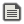Nintendo shutting down 3DS + Wii U online services, see our post

# Clipping with camera in 3DVakoreCreated:This is pretty general, but how would I perform camera clipping in a 3d space? I know how to do it in 2d, but 3d is causing me issues. For 2D clipping, I used the Cohen Sutherland algorithm. Anyone know how I can extend this into 3d? NOTE: This is what my projection code is(from 3d to 2d to project onto screen) var project = function(vert) { var x = vert; var y = vert; var z = vert; var rY = rotate2d(x - camPos.x, z - camPos.z, camPos.ry * Math.PI / 180); x = rY; z = rY; var rX = rotate2d(y - camPos.y, z, camPos.rx * Math.PI / 180); y = rX; z = rX; f = 455 / z; x = x * f; y = y * f; return {x:x,y:y,z:z}; }; In short, what I am trying to do is prevent 'z' from being less than 0(can't just constrain it, that causes problems), as that is what causes everything behind it to go kabloo-y(yes, I have code that checks if three triangle points are behind the camera to prevent drawing in that case). What I currently need is a formula to make 'x' and 'y' what they would be when Z is 0, without overflowing(if that makes sense). Also, don't worry about rotate2d, that is just a function( I took from here: https://www.youtube.com/watch?v=g4E9iq0BixA Just assume camera movement I guess(I'm pretty sure I'm confusing you guys). Thanks in advance!I don't get what you mean by 3D clipping. Are you blocking the screen for UI or something, or are you just hiding some of the bg elements?Figured it out: var intersect3d = function(vert, vert2) { var x = vert; var y = vert; var z = vert; var rY = rotate2d(x - camPos.x, z - camPos.z, camPos.ry * Math.PI / 180); x = rY; z = rY; var rX = rotate2d(y - camPos.y, z, camPos.rx * Math.PI / 180); y = rX; z = rX; var x2 = vert2; var y2 = vert2; var z2 = vert2; var rY2 = rotate2d(x2 - camPos.x, z2 - camPos.z, camPos.ry * Math.PI / 180); x2 = rY2; z2 = rY2; var rX2 = rotate2d(y2 - camPos.y, z2, camPos.rx * Math.PI / 180); y2 = rX2; z2 = rX2; x = x + (x2 - x) * (0.1 - z) / (z2 - z); y = y + (y2 - y) * (0.1 - z) / (z2 - z); z = 0.1; f = 455 / z; x = x * f; y = y * f; return {x:x,y:y,z:z}; }; Anyone can use this without creditting me, though I got some formulas from the video, so maybe credit the creator of itnI don't get what you mean by 3D clipping. Are you blocking the screen for UI or something, or are you just hiding some of the bg elements?
3D clipping is somehow a field of view in terms of near/far to prevent stuff between the actor and the camera from blocking in the remderI don't get what you mean by 3D clipping. Are you blocking the screen for UI or something, or are you just hiding some of the bg elements?
3D clipping is somehow a field of view in terms of near/far to prevent stuff between the actor and the camera from blocking in the remder
Oh okBtw thanks for the video link! I understand how to code this method more than the triangle method thanks to this! EDIT: I realize now that this is probably what everyone uses, but triangles just connect the vertices lol